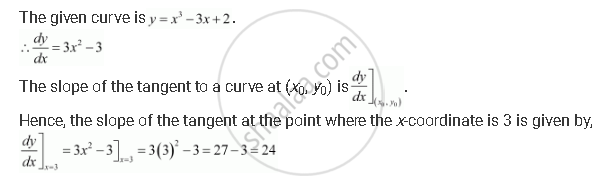Share

# Find the Slope of the Tangent to the Curve Y = X3 − 3x + 2 at the Point Whose X-coordinate is 3. - CBSE (Commerce) Class 12 - Mathematics

#### Question

Find the slope of the tangent to the curve y = x3 − 3x + 2 at the point whose x-coordinate is 3.

#### SolutionIs there an error in this question or solution?

#### APPEARS IN

NCERT Solution for Mathematics Textbook for Class 12 (2018 to Current)
Chapter 6: Application of Derivatives
Q: 4 | Page no. 211

#### Video TutorialsVIEW ALL 

Solution Find the Slope of the Tangent to the Curve Y = X3 − 3x + 2 at the Point Whose X-coordinate is 3. Concept: Tangents and Normals.
S